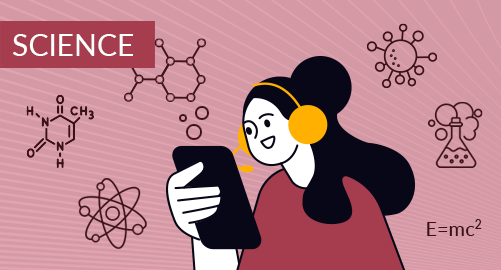#### through a demo today# Year 10 Science

Science
Year 10

The Year 10 Science curriculum compartmentalised as biological sciences for scientific findings related to living things, physicochemical sciences with data analysis, research skills, experimental techniques, and Earth sciences to study global systems.

## What you'll learn

• 1

Genetics

• 2

Evolution

• 3

The periodic table

• 4

Chemical reaction

• 5

Global systems

• 6

The universe

• 7

Motion

• 8

Force, work, and energy

## Skills you'll learnExperimental design, analytical skills - Molecular biology tests, pedigree analysis, genome sequencing, gene techniques, genetic testing, human genetics, medical genetics, and counsellingCritical thinking and observation skills - Phylogenetics, palaeontology, molecular evolution, adaptationsAnalysing skills - Element identification, structure of atom, elemental study, types of bonds, periodic trends, metals, non-metals, transition metals, noble gases, periodic table applicationsChemical analysis - Balancing an equation, types of chemical reaction, stoichiometry, chemical kinetics, reaction mechanism, reaction energetics, chemical safety measures, reaction rate analysisObservation skills - Earth's structure, geological study, plate tectonics, climatic systems, relation between biogeochemical cycles, remedial measures to control human impactResearch skills - Theory of relativity, gravitational waves, astronomical observations, elements of universe, cosmological researchQuantitative analysis skills - Kinematics, equations of motion, position-time graphs, velocity-time graphs, applications of Newton's laws, calculating force equation, mathematical modelling, dimensional analysis, GPSProblem solving skills - Types of forces, characteristics of forces, mathematical description of force, knowledge on applied force, calculating energy, calculating kinetic energy, calculating systems, and efficiency